×#### Thank you for registering.

One of our academic counsellors will contact you within 1 working day.

Click to Chat

1800-1023-196

+91-120-4616500

CART 0

• 0

MY CART (5)

Use Coupon: CART20 and get 20% off on all online Study Material

ITEM
DETAILS
MRP
DISCOUNT
FINAL PRICE
Total Price: Rs.

There are no items in this cart.
Continue Shopping• Complete Physics Course - Class 11
• OFFERED PRICE: Rs. 2,968
• View Details

```Doppler Effect

The whistle of a fast moving train appears to increase in pitch as it approaches a stationary observer and it appears to decrease as the train moves away from the observer. This apparent change in frequency was first observed and explained by Doppler in 1845.

The phenomenon of the apparent change in the frequency of sound due to the relative motion between the source of sound and the observer is called Doppler effect.

The apparent frequency due to Doppler effect for different cases can be deduced as follows.

(i) Both source and observer at rest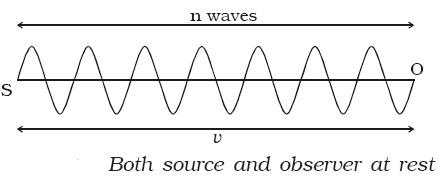Suppose S and O are the positions of the source and the observer respectively. Let n be the frequency of the sound and v be the velocity of sound. In one second, n waves produced by the source travel a distance SO = v  (Fig. 7.19a).

The wavelength is λ = n

(ii) When the source moves towards the stationary observer

If the source moves with a velocity vs  towards the stationary observer, then after one second, the source will reach S’, such that SS’ = vs. Now n waves emitted by the source will occupy a distance of (v – vs) only as shown in figure.

Therefore the apparent wavelength of the sound is

λ’ = v – vs/n

The apparent frequency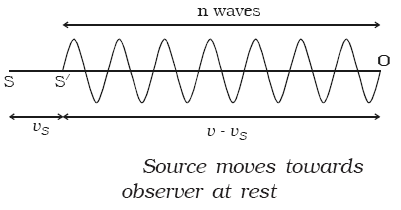n’ = v/λ’ = (v/v – vs)n             …... (1)

So,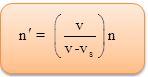As n′ > n, the pitch of the sound appears to increase.

When the source moves away from the stationary observer

If the source moves away from the stationary observer with velocity vs, the apparent frequency will be given by

n’ = [v/v – (-vs)]n = [v/v + vs] n         …... (2)

So,As n′ < n, the pitch of the sound appears to decrease.

(iii) Source is at rest and observer in motion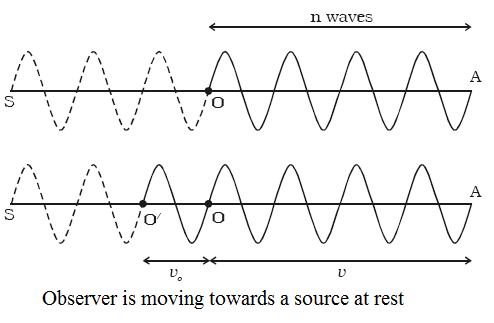S and O represent the positions of source and observer respectively.

The source S emits n waves per second having a  wavelength λ = v/n

Consider a point A such that OA contains n waves which crosses the ear of the observer in one second (Fig. 7.20a). (i.e) when the first wave is at the point A, the nth wave will be at O, where the observer is situated.

When the observer moves towards the stationary source,

Suppose the observer is moving towards the stationary source with velocity vo. After one second the observer will reach the point O′ such that OO′ = vo. The number of waves crossing the observer will be n waves in the distance OA in addition to the number of waves in the distance OO′ which is equal to  v0/λ as shown in Fig. 7.20b. Therefore, the apparent frequency of sound is

n’ = n + v0/λ = n + (v0/v) n

So,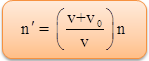…... (3)

As n’ > n, the pitch of the sound appears to increase.

When the observer moves away from the stationary source,

n’ = [v + (-v0)/v] n

So,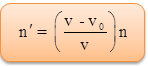…... (4)

As n′ < n, the pitch of sound appears to decrease.

Note : If the source and the observer move along the same direction, the equation for apparent frequency is,…... (5)

Suppose the wind is moving with a velocity W in the direction of propagation of sound, the apparent frequency is

n’ = (v +W– v0/v +W– vs) n                …... (6)

Refer this video to know more about on Doppler effect

Applications of Doppler effect

(a) To measure the speed of an automobile

An electromagnetic wave is emitted by a source attached to a police car. The wave is reflected by a moving vechicle, which acts as a moving source. There is a shift in the frequency of the reflected wave. From the frequency shift using beats, the speeding vehicles are trapped by the police.

(b) Tracking a satellite

The frequency of radio waves emitted by a satellite decreases as the satellite passes away from the Earth. The frequency received by the Earth station, combined with a constant frequency generated in the station gives the beat frequency. Using this, a satellite is tracked.

A RADAR sends high frequency radiowaves towards an aeroplane. The reflected waves are detected by the receiver of the radar station. The difference in frequency is used to determine the speed of an aeroplane.

(d) Sonar (Sound navigation and ranging)

Sound waves generated from a ship fitted with SONAR are transmitted in water towards an approaching submarine. The frequency of the reflected waves is measured and hence the speed of the submarine is calculated.An approaching source moves closer during period of the sound wave so the effective wavelength is shortened, giving a higher pitch since the velocity of the wave is unchanged.

The speed of sound is determined by the medium in which it is traveling, and therefore is the same for a moving source.

The Doppler effect is observed whenever the source of waves is moving with respect to an observer.

For the same period of time, the same number of waves must fit between the source and the observer.

If the source is moving towards the observer, the observer perceives sound waves reaching him or her at a more frequent rate (high pitch).

If the source is moving away from the observer, the observer perceives sound waves reaching him or her at a less frequent rate (low pitch).

The Doppler effect can be described as the effect produced by a moving source of waves in which there is an apparent upward shift in frequency for observers towards whom the source is approaching and an apparent downward shift in frequency for observers from whom the source is receding.

The Doppler effect can be observed for any type of wave - water wave, sound wave, light wave, etc.

The Doppler effect - an apparent shift in frequency for a sound wave produced by a moving source.

The Doppler effect is of intense interest to astronomers who use the information about the shift in frequency of electromagnetic waves produced by moving stars in our galaxy and beyond in order to derive information about those stars and galaxies.

Problem (JEE Main):

Two sound sources are moving in opposite directions with velocities v1 and v2 ( v1 > v2). Both are moving away from a stationary observer. The frequency of both the sources is 900 Hz. What is the value of v1 – v2 so that the beat frequency observed by the observer is 6 Hz. Speed of sound v = 300 m/s. Given, that v1 and v2 « v.

(a) 1 m/s                      (b) 2 m/s

(c) 3 m/s                       (d) 4 m/s

Solution:

f1 = 900 (300/300+v1) = 900 (1+v1/300)-1 ≈ 900 – 3v1

Similarly

f2 = 900 (300/300+v2) = 900 – 3v2

f2 – f1 = 6

So, 3 (v1 – v2) = 6

Or, v1 – v2 = 2 m/s

Thus, from the above observation we conclude that, option (b) is correrct.Question 1

The number of beats produced per second is equal to

(a) the sum of the frequencies of two tuning forks

(b) the difference of the frequencies of two tuning forks

(c) the ratio of the frequencies of two tuning forks

(d) the frequency of either of the two tuning forks

Question 2

Doppler effect applies to

(a) sound wave only                               (b) light wave only

(c) both sound and light waves               (d) neither sound nor light wave

Question 3

Silence zone takes place due to

(a) constructive interference             (b) destructive interference

(c) beats                                            (d) resonance

Question 4

The speed of stationary waves in a stretched string are independent of

(a) Number of loops                              (b) Tension in the string

(c) Point where string is plucked          (d) both (a) and (c)

Question 5

Which phenomena can be applied to estimate the velocity of star with respect to earth

(a) Dopplers effect                  (b) Interference of waves

(c) Beats phenomena             (d) All of theseQ.1
Q.2
Q.3
Q.4
Q.5

b

c

b

d

a

Related Resources:-

You might like to magnification and thin lens.

For getting an idea of the type of questions asked, refer the  Previous Year Question Papers.

To read more, Buy study material of Waves comprising study notes, revision notes, video lectures, previous year solved questions etc. Also browse for more study materials on Physics here.

```### Course Features

• 101 Video Lectures
• Revision Notes
• Previous Year Papers
• Mind Map
• Study Planner
• NCERT Solutions
• Discussion Forum
• Test paper with Video Solution